# Six Sigma Online

## Performing a Long-Term MSA Study

Ahh, measurement system analysis—the basis for all our jobs because, as Lord Kelvin said, “… When you cannot measure it, when you cannot express it in numbers, your knowledge is of a meager and unsatisfactory kind.” How interesting it is then, that we who thrive on data so frequently don't have any proof that the numbers we're using relate to the event we are measuring—hence my past few articles about the basics of measurement system analysis in “Letting You In on a Little Secret,” on how to do a potential study in “The Mystery Measurement Theatre, and on how to do a short-term study in “Performing a Short-Term MSA Study.” The only (and most important) topic remaining is how to perform a long-term study, which is the problem I left with you last month.

So read on to see how.

The potential study told us if we even have a hope of being able to use a measurement system to generate data (numbers related to an event) as opposed to numbers (symbols manipulated by mathematicians for no apparent reason other than entertainment). The short-term study allowed us to test the system performance a little more rigorously, perhaps in preparation for using it in our process. A measurement system’s performance is quantified by:

• Repeatability—the amount of variability the same system (operator, device) exhibits when measuring exactly the same thing multiple times
• Reproducibility—the amount of variability due to different operators using the same device, or maybe the same operators using different devices
• %R&R—a combination of the repeatability and reproducibility that tells us how easy it is for this measurement system to correctly classify product as conforming or nonconforming to our specification
• Bias—the amount that the average measurement is off of the “true” value

However, none of these things have any meaning if the measurement system changes through time, and neither the potential nor short-term study really tests for through-time stability. With an unstable gauge, I might convene a Six Sigma team to work on a problem that doesn’t exist, put in systems to control a process based on a random number generator, or scrap product that is conforming. Simply put, my ability to understand and control my process is completely compromised.  A measurement system that is out of control is even worse than a process that is out of control, since we don’t even have a hint of what is really going on.

Thus the need for the long-term study, which allows us to assess in detail exactly how our measurement system is performing through time. The long-term study is the Holy Grail (not the Money Python kind) of measurement system analysis, and with it we can state with confidence that the system is producing data, and not just numbers.

As before, I gave you a link to a totally free and awesome spreadsheet that will help you with your MSA work, and I gave you some data for the following scenario:

A statistical facilitator and an engineer wish to conduct a gauge capability analysis (long-term) for a particular ignition signal processing test on engine control modules. The test selected for study measures voltage which has the following specifications:

IGGND = 1.4100 ± 0.0984 Volts (Specification)

Eight control modules are randomly selected from the production line at the plant, and run (in random order, of course) through the tester at one hour intervals (but randomly within each hour). This sequence is repeated until 25 sample measures (j = 25) of size eight (n = 8) have been collected. The assumption is that these voltages are constant and the only variation we see in remeasuring a module is gauge variation.

Right off the bat, we know that we only measure this engine control module; if we measured others or to different target voltages, we would include them in the study as well. We select these eight parts and keep remeasuring the same ones each hour. We also are assuming that different operators have no effect, since we are only using one.

To start off the spreadsheet calculates the mean and standard deviation across each hour’s measurements. Regardless of the actual voltages the eight modules have, the average of those voltages must be the same, right? One way we will eventually look at the measurement error over time will be by looking at how that average moves around. Because we have eight modules, we can also calculate a standard deviation across these eight. But be careful that you understand what this is. There are two components of variability in this standard deviation, only one of which relates to gauge variability. There is some measurement error as I take a reading for each one, but there is also the fact that the eight modules are producing somewhat different voltages from each other as well. Even if there were no measurement error, we still would calculate a standard deviation due to the part differences. This second variance component is of no interest to us for the MSA, but we had better not forget about it as we go forward.Figure 1: Worksheet calculations

First, we need some validation that we can use the numbers. If the measurement process is in control, then the measurements for each part over time should be in control. So we take a look at each part on an individuals chart (the limit comes from the moving ranges, which I leave out of sight for this example). In order for the moving range to relate to the dispersion, we want to check normality for all the parts: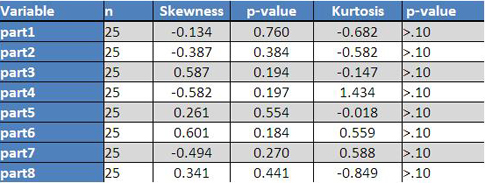Figure 2: Normality tests for the eight modules—output from MVP stats

With a sample size of 25 we probably are going to rely on the skewness and kurtosis indices, and they allow us to assume the variability is distributed normally. So let’s take a look at those individual charts on all the parts.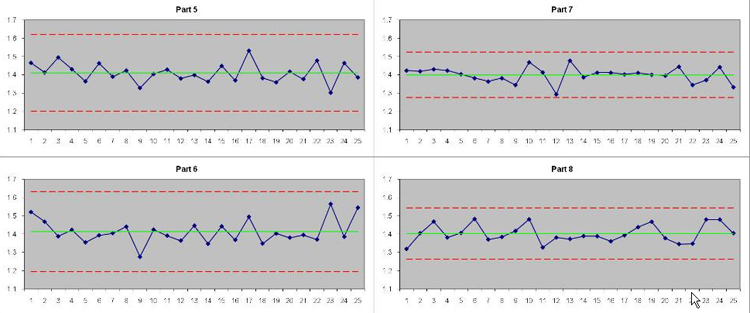Figure 3: Individuals charts for each part through time—limits from the moving range

We do see a point outside the lower control limit on part 4 and a larger than expected moving range on part 7 (both of which we would have investigated once the long-term study was in place). But two out of 200 observations is well within what we would expect with Type I error rate of 0.0027 (the rate you get a point out of the ±3σ control limits due to chance and chance alone) so I am comfortable saying that, so far, the gauge looks stable with respect to location. If a particular part became damaged at some point, or was a lot more difficult to read than another part, it should show up on these charts.

While individual charts are really useful, they have their weaknesses, one of which is a lack of sensitivity to pretty big shifts in the mean. Thankfully, we have those means we calculated back in the worksheet calcul figure 1 to increase the sensitivity to a global shift in the average. Remember how the standard deviation across the parts has two components of variability? That is why we can’t just do an X-bar and an s chart using the usual limits—that “s” is inflated due to the part-to-part differences and will give us limits on the mean and the s chart that are too large. We get around this by recalling that we can plot any statistic on an individuals chart—though we may have to adjust the limits for the shape of the distribution. We are in good shape (pun intended) to use the individuals chart for our means, because due to the central limit theorem, those 25 averages of eight modules will tend to be distributed normally, and therefore the moving range of the means will relate to the dispersion of the means.Figure 4: Means as individuals control chart—limits from the moving range

Figure 4 further supports our notion that the location measurements are stable through time. If there were some sort of a shift across many or all the parts, it would show up here, so actions such as a recalibration, retaring, or dropping the gauge on the floor would show up on this chart. Due to the central limit theorem, this chart will be far more sensitive to shifts in the average than the individuals charts on each part.

We also know that control for continuous data isn't assessed by just looking at the average—we need to look at that dispersion as well. Using the same trick as with the means, we will plot the standard deviations (extra component of variability and all) on an individuals chart. If the measurement error is normal and the same for every part, then regardless of the actual voltages the standard deviations across the parts ought to be distributed close to (though not exactly) normal. (Again, this is different than the random sampling distribution of the standard deviation, which would be very clearly positively skewed.)

The upshot is that we plot the standard deviations on an individuals chart with limits from the moving ranges as well.Figure 5: Standard deviations across eight parts plotted as individuals—limits from the moving range

Here we have one point outside of the limits, which if we were up and running with the chart, we would have reacted to by investigating. Because the measurements are already done, I am going to continue watching that control chart very closely and any statement about control is going to be provisional. The types of events that would show up here would be if there was a global change in the dispersion of the measurements—perhaps a control circuit going bad or a change in the standard operating procedure.

As with the short-term study, we also want to keep an eye on the relationship between the average measurement and the variation of the readings. We want to see no correlation between them—if we do, then the error of the measurement changes with the magnitude of what you are measuring, and so your ability to correctly classify as conforming or not changes too. We will check that with a correlation between the mean and standard deviation.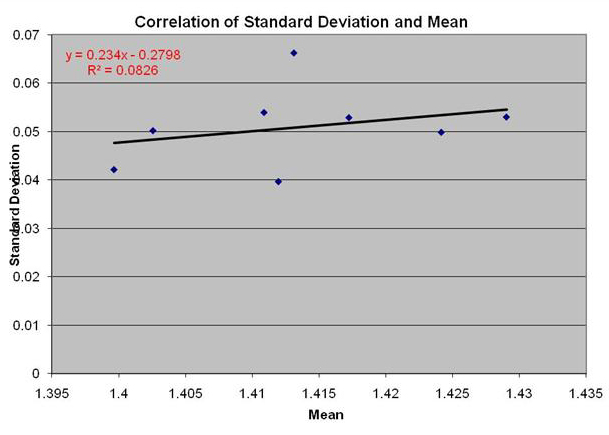Figure 6: Correlation between magnitude (mean) and variation (standard deviation)

We only have eight points because we only have eight different parts. Normally, we won’t do correlations on such few points, but we would have already tested this with the short-term study. (Which you DID do, right?) This is just to make sure nothing really big changed. This correlation is not significant, so we can check that off our list.

At this point we are (provisionally) saying that there is nothing terribly strange going on in our measurement system through time—it seems to be stable sliced a couple of ways, and the magnitude and dispersion are independent. We can now, finally, begin to answer our question about the repeatability and reproducibility. In our case, we only have one operator and system, so measurement error due to reproducibility is assumed to be zero. If we had tested multiple operators, the estimate of the variability due to operator would be:Which is pretty similar to that formula you remember from SPC. The range across the operator averages divided by our old friend d2 on the expanded d2 table with g = 1 and m = the number of appraisers. The spreadsheet is set up to handle up to two operators.

All we are looking at (in this case, to estimate) is the variability due to repeatability, which is: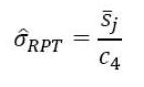You recognize that from SPC as well I bet. The only difference is that we are taking the average of the standard deviations for each of the j = 8 modules and dividing by c4 for the number of measurements (25 here). The spreadsheet cleverly does all this for you.Figure 7: Spreadsheet output

Again, we are interested in the ability of this device to correctly categorize whether a given module is in or out of spec. Our spec width was 0.1968V, and so we put that into the %R&R formula:We find that the measurement error alone takes up 134.74 percent of the spec.

Uh-oh. What is it with these measurement devices?

If I measure a part that is smack dab in the middle of the spec over time, I would see this distribution: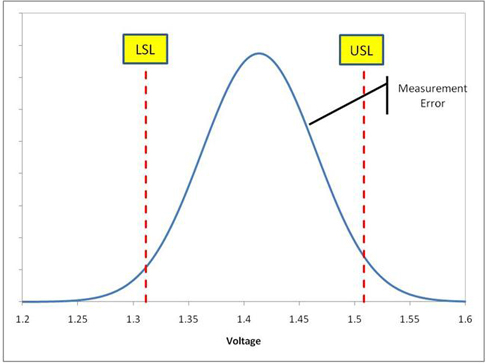Figure 8: Measurement error

That means that on any individual measurement (how we have been using this gauge up to this point) of a module that is exactly on target at 1.41, I could reasonably see a reading somewhere between 1.26 and 1.56—in or out of spec at the whim of the gauge variability.

Do you remember that crazy graph that showed the probability of incorrectly classifying a part as conforming or nonconforming on a single measure? Here it is for our voltage measurement system: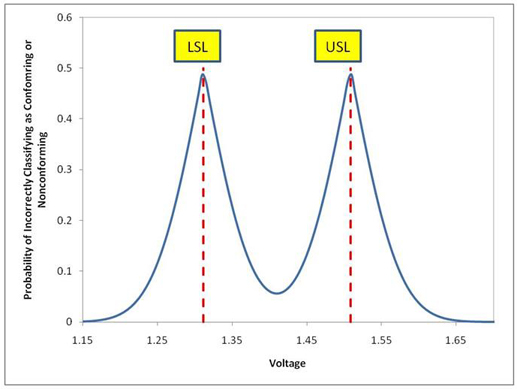Figure 9: Probability of incorrectly classifying module conformance on a single measurement

Once again, we have a measurement system that is probably no good for making conformance decisions on a single measurement. It is stable through time, yes, but so highly variable that we stand a pretty good chance of calling good stuff bad and bad stuff good.

Because it's stable, we could conceive of taking multiple measurements to use the average of those readings to determine conformance:

Say we are looking for a  %R&R of 10 percent: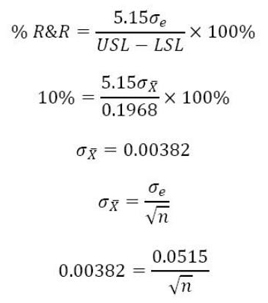Leaving us measuring the voltage 182 times to get that %R&R.

Ahh, that’s not gonna happen.

We need to figure out what is causing all the variability in this measurement device, or replace it with something that is capable of determining if a module is in conformance with the spec. It is also possible that the modules themselves are contributing noise to the measurements—maybe our assumption that they give the same voltage time after time is wrong. That would be a good thing to find out, too.

We have some more work to do, it seems.

Note that we did not assess bias—with this gauge, it would be a waste of time since it is unusable for this specification. If we wanted to, all we have to do is get the “true” voltages of those eight modules, get the average of the true values, and see how far off that average is from our measured average. If they are statistically different, we just add or subtract the amount of bias to get an accurate (but not precise with this system) measurement. You would want to track this bias with time on an control chart as well, to make sure it was stable too.

If you have been reading these mini-dissertations on MSA, you will know that a common assumption of them all is that the measurement is not destructive—that what you are measuring remains constant with time. What do you do if that is not the case?

You read next month’s column.

### Random Heresy

#### Six Sigma in Hard Economic Times

What purpose, if any, does Six Sigma serve in economic downturns? Full disclosure: I teach and consult in Six Sigma and related areas, and you’re reading this article because you’re interested in Six Sigma, so we may not be the most objective people to assess this, but in this article, I w

...

### News Flash

Six Sigma's lead instructor Steven Ouellette wrote an article with Dr. Jeffrey Luftig on "The Decline of Ethical Behavior in Business."

Six Sigma Online's lead instructor Steven Ouellette was profiled in the June 2008 issue of Quality Digest magazine. If you want to learn more about Steve's peculiar view of the world, as well as what he studied for a year in Europe, read the profile online.